# IoT | TensorFlow Weather Station Predicts Rainfall Intensity

Collates local weather data on Google Sheets and interprets it with a neural network model built w/ TensorFlow to predict rainfall intensity

ExpertFull instructions provided5 hours9,712

## Things used in this project

### Hardware components

 DFRobot Weather Station Kit with Anemometer/Wind Vane/Rain Bucket
×1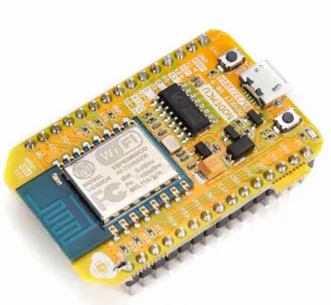NodeMCU ESP8266 Breakout Board ESP-12E
×1
 DFRobot SIM808 GPS/GPRS/GSM Shield For Arduino
×1Arduino UNO
×1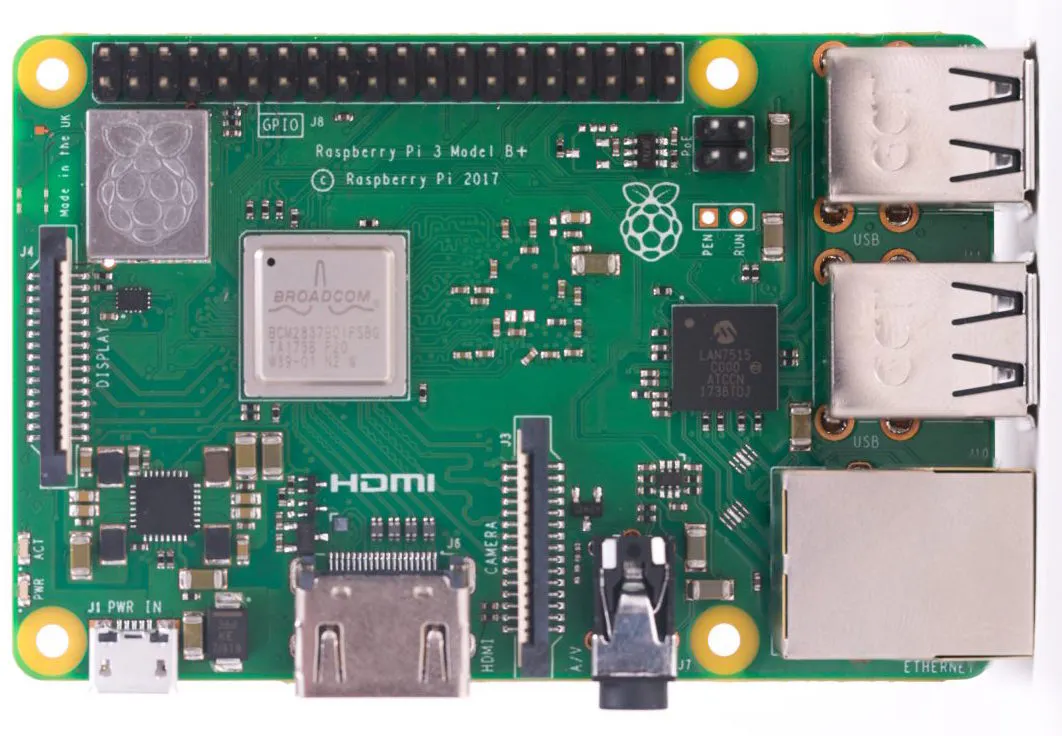Raspberry Pi 3 Model B+ Raspberry Pi 3B+ or 4
×1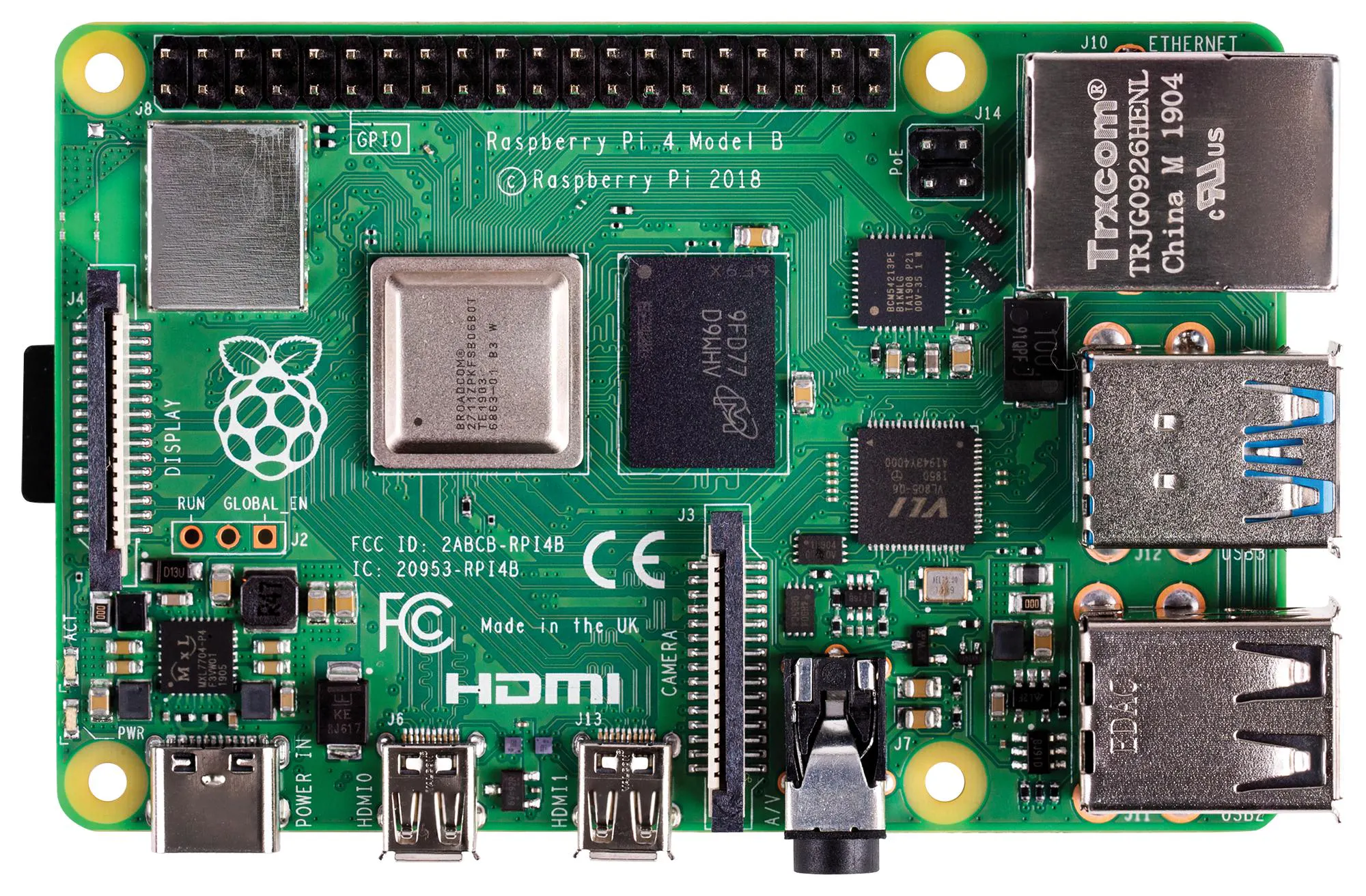Raspberry Pi 4 Model B Raspberry Pi 3B+ or 4
×1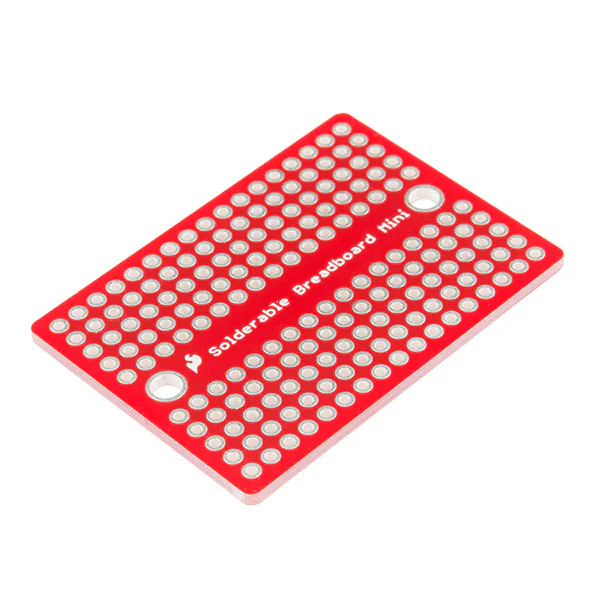×2

### Software apps and online servicesArduino IDETensorFlowMicrosoft Visual Studio 2017

### Hand tools and fabrication machinesHot glue gun (generic)

## Schematics

### Schematic-1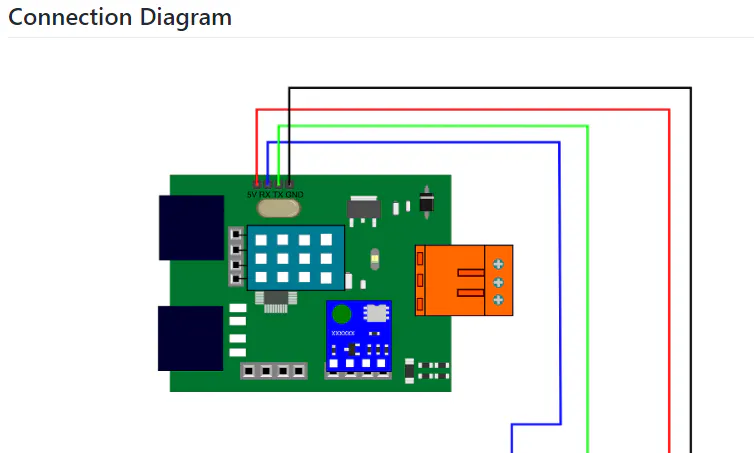### Schematic-2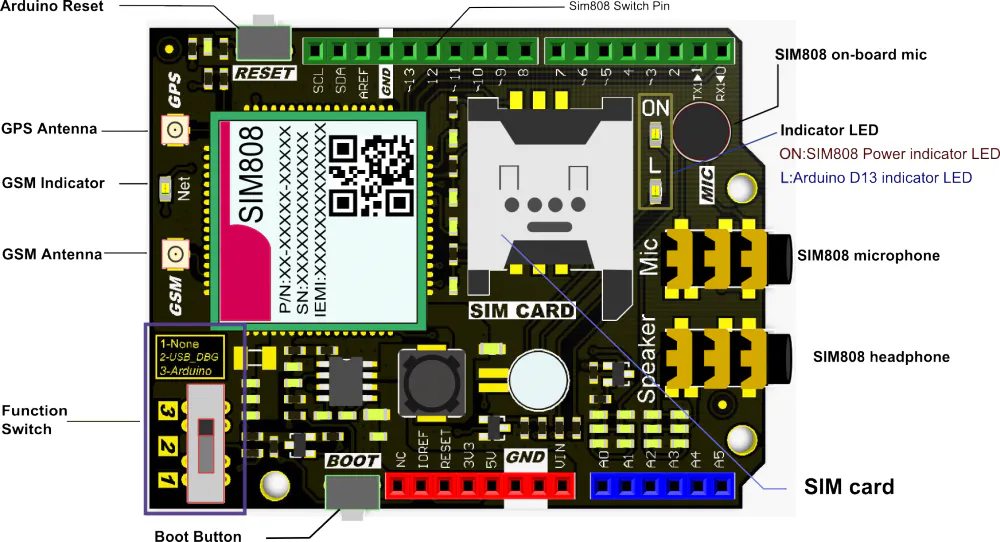## Code

### IoT_weather_station_neural_network.py

Python
```# IoT Weather Station Predicting Rainfall Intensity w/ TensorFlow

# Windows, Linux, or Ubuntu

# By Kutluhan Aktar

# Collates weather data on Google Sheets and interprets it with a neural network built in TensorFlow to make predictions on the rainfall intensity.

# https://www.theamplituhedron.com/projects/IoT_Weather_Station_Predicting_Rainfall_Intensity_with_TensorFlow

import tensorflow as tf
from tensorflow import keras
import matplotlib.pyplot as plt
import numpy as np
import pandas as pd

# Create a class to build a neural network after getting, visualizing, and scaling (normalizing) weather data.
class Weather_Station:
def __init__(self, data):
self.df = data
self.input = []
self.label = []
# Define class names for different rainfall intensity predictions and values.
self.class_names = ['None', 'Light Rain', 'Moderate Rain', 'Heavy Rain', 'Violent Rain']
# Create graphics for requested columns.
def graphics(self, column_1, column_2, xlabel, ylabel):
# Show requested columns from the data set:
plt.style.use("dark_background")
plt.gcf().canvas.set_window_title('IoT Weather Station')
plt.hist2d(self.df[column_1], self.df[column_2])
plt.colorbar()
plt.xlabel(xlabel)
plt.ylabel(ylabel)
plt.title(xlabel)
plt.show()
# Visualize data before creating and feeding the neural network model.
def data_visualization(self):
# Inspect requested columns to build a model with appropriately formatted data:
self.graphics('WD', '1h_RF', 'Wind Direction (deg)', 'One-Hour Rainfall (mm)')
self.graphics('Av_WS', '1h_RF', 'Average Wind Speed (m/s)', 'One-Hour Rainfall (mm)')
self.graphics('Mx_WS', '1h_RF', 'Maximum Wind Speed (m/s)', 'One-Hour Rainfall (mm)')
self.graphics('24h_RF', '1h_RF', '24-Hour Rainfall (mm)', 'One-Hour Rainfall (mm)')
self.graphics('Tem', '1h_RF', 'Temperature (C)', 'One-Hour Rainfall (mm)')
self.graphics('Hum', '1h_RF', 'Humidity (%)', 'One-Hour Rainfall (mm)')
self.graphics('b_PR', '1h_RF', 'Barometric Pressure (hPA)', 'One-Hour Rainfall (mm)')
# Scale (normalize) data depending on the neural network model.
def scale_data(self):
# Wind Direction and Speed:
wv = self.df.pop('Av_WS')
max_wv = self.df.pop('Mx_WS')
# Calculate the wind x and y components.
# Calculate the max wind x and y components.
# Temperature:
tem = self.df.pop('Tem')
self.df['scaled_Tem'] = tem / 25
# Humidity:
hum = self.df.pop('Hum')
self.df['scaled_Hum'] = hum / 70
# Barometric Pressure:
bPR = self.df.pop('b_PR')
self.df["scaled_bPR"] = bPR / 1013
# 24 Hour Rainfall (Approx.)
rain_24 = self.df.pop('24h_RF')
self.df['scaled_24h_RF'] = rain_24 / 24
# Define the input and label arrays.
def create_input_and_label(self):
n = len(self.df)
# Create the input array using the scaled variables:
for i in range(n):
self.input.append(np.array([self.df['scaled_WX'][i], self.df['scaled_WY'][i], self.df['scaled_max_WX'][i], self.df['scaled_max_WY'][i], self.df['scaled_Tem'][i], self.df['scaled_Hum'][i], self.df['scaled_bPR'][i]]))
self.input = np.asarray(self.input)
# Create the label array using the one-hour and 24-hour rainfall variables:
for i in range(n):
_class = 0
# Evaluate the approximate rainfall rate:
approx_RF_rate = (self.df['1h_RF'][i] + self.df['scaled_24h_RF'][i]) * 100
# As labels, assign classes of rainfall intensity according to the approximate rainfall rate (mm):
if approx_RF_rate == 0:
_class = 0
elif approx_RF_rate < 2.5:
_class = 1
elif 2.5 < approx_RF_rate and approx_RF_rate < 7.6:
_class = 2
elif 7.6 < approx_RF_rate and approx_RF_rate < 50:
_class = 3
else:
_class = 4
self.label.append(_class)
self.label = np.asarray(self.label)
# Split the data for the training and test sets.
def split_data(self):
n = len(self.df)
# (60%, 40%) - (training, test)
self.train_input = self.input[0:int(n*0.6)]
self.test_input = self.input[int(n*0.6):]
self.train_label = self.label[0:int(n*0.6)]
self.test_label = self.label[int(n*0.6):]
# Build and train the artificial neural network (ANN) to make predictions on the rainfall intensity with classes.
def build_and_train_model(self):
# Build the neural network:
self.model = keras.Sequential([
keras.Input(shape=(7,)),
keras.layers.Dense(16, activation='relu'),
keras.layers.Dense(32, activation='relu'),
keras.layers.Dense(64, activation='relu'),
keras.layers.Dense(128, activation='relu'),
keras.layers.Dense(5, activation='softmax')
])
# Compile:
# Train:
self.model.fit(self.train_input, self.train_label, epochs=19)
# Test the accuracy:
print("\n\nModel Evaluation:")
test_loss, test_acc = self.model.evaluate(self.test_input, self.test_label)
print("Evaluated Accuracy: ", test_acc)
# Make rainfall intensity class [0 - 4] predictions using different input arrays.
def make_prediction(self, pre_array):
print("\n\nModel Predictions:\n")
prediction = self.model.predict(pre_array)
for i in range(len(pre_array)):
print("Prediction => ", self.class_names[np.argmax(prediction[i])])
# Save the model for further usage without training steps:
def save_model(self):
self.model.save("E:\PYTHON\weather_station.h5")
# Run Artificial Neural Network (ANN):
def Neural_Network(self, save):
self.scale_data()
self.create_input_and_label()
self.split_data()
self.build_and_train_model()
if save == True:
self.save_model()
# Example Input and Layer:
print("\nScaled Input [EXP]:\n")
print(self.train_input)
print("\nScaled Label [EXP]:\n")
print(self.train_label)

# Read data (Remote Weather Station.csv):
csv_path = "E:\PYTHON\Remote Weather Station.csv"

# Define a new class object named 'station':
station = Weather_Station(df)

# Visualize data:
#station.data_visualization()

# Artificial Neural Network (ANN):
station.Neural_Network(False)

# Enter inputs for making predictions:
prediction_array = np.array([
[0, 0, 0.31819805, 0.31819805, 0.6988, 0.81498571, 0.99349753],
[0, -0, 0, -0, 0.8444, 1, 0.96835143],
[0, 0, 0.45, 0, 0.87577, 0.95857143, 1.00128332],
[-0, -0, -0, -0, 0.8224, 1.05714286, 0.99279368]
])
# Prediction Results:
station.make_prediction(prediction_array)
```

### ESP12E_remote_weather_station.ino

Arduino
```         /////////////////////////////////////////////
//    IoT Weather Station Predicting       //
//    Rainfall Intensity w/ TensorFlow     //
//         ------------------------        //
//           NodeMCU (ESP-12E)             //
//           by Kutluhan Aktar             //
//                                         //
/////////////////////////////////////////////

//
// Collates weather data on Google Sheets and interprets it with a neural network built in TensorFlow to make predictions on the rainfall intensity.
//
// https://www.theamplituhedron.com/projects/IoT_Weather_Station_Predicting_Rainfall_Intensity_with_TensorFlow
//
// Connections
// NodeMCU (ESP-12E) :
//                                Weather Station
// VV  --------------------------- 5V
// D5  --------------------------- RX
// D6  --------------------------- TX
// G   --------------------------- GND

// Include required libraries:
#include <ESP8266WiFi.h>
#include <WiFiClient.h>
#include <ESP8266WebServer.h>
#include <ESP8266HTTPClient.h>
#include <SoftwareSerial.h>

const char *ssid = "<SSID>";

// Define weather station settings:
char databuffer;
double temp;
int transferring = 0;

// Define the serial connection pins - RX and TX.
SoftwareSerial Serial_1(D6, D5); // (Rx, Tx)

void setup() {
// Wait until connected.
delay(1000);
// Initiate serial ports:
Serial.begin(115200);
Serial_1.begin(9600);
// It is just for assuring if connection is alive.
WiFi.mode(WIFI_OFF);
delay(1000);
// This mode allows NodeMCU to connect any WiFi directly.
WiFi.mode(WIFI_STA);
// Connect NodeMCU to your WiFi.

Serial.print("\n\n");
Serial.print("Try to connect to WiFi. Please wait! ");
Serial.print("\n\n");
// Halt the code until connected to WiFi.
while (WiFi.status() != WL_CONNECTED) {
delay(500);
Serial.print("*");
}

// If connection is successful:
Serial.print("\n\n");
Serial.print("-------------------------------------");
Serial.print("\n\n");
Serial.print("Connection is successful!");
Serial.print("\n\n");
Serial.print("Connected WiFi SSID : ");
Serial.print(ssid);
Serial.print("\n\n");
Serial.println(WiFi.localIP());
Serial.print("\n\n");

}

void loop() {
// Get data from the remote weather station:
getBuffer();
// Debug the information and create the link:
String weather_data = "wd=" + String(WindDirection()) + "&a_ws=" + String(WindSpeedAverage()) + "&m_ws=" + String(WindSpeedMax()) + "&1_rf=" + String(RainfallOneHour()) + "&24_rf=" + String(RainfallOneDay()) + "&tem=" + String(Temperature()) + "&hum=" + String(Humidity()) + "&b_pr=" + String(BarPressure());
String server = "http://192.168.1.24/remote_weather_station/?";
Serial.println("Weather Data => " + weather_data);
Serial.println("Buffer => " + String(databuffer));
// Send data packets every 5 minutes to Raspberry Pi (or any server).
transferring++; Serial.println("Time => " + String(transferring) + "s / " + String(int(5*60)) + "s\n\n");
if(transferring == 5*60){
// Create the HTTP object to make a request to the server.
HTTPClient http;
http.begin(server + weather_data);
int httpCode = http.GET();
Serial.println("Data Send...\nHTTP Code => " + String(httpCode) + "\nServer Response => " + payload + "\n\n");
http.end();
transferring = 0;
}
// Wait 1 second...
delay(1000);
}

// WEATHER STATION
void getBuffer(){
int index;
for (index = 0;index < 35;index ++){
if(Serial_1.available()){
if (databuffer != 'c'){
index = -1;
}
}
else{
index --;
}
}
}

int transCharToInt(char *_buffer, int _start, int _stop){
int _index;
int result = 0;
int num = _stop - _start + 1;
int _temp[num];
for (_index = _start;_index <= _stop;_index ++){
_temp[_index - _start] = _buffer[_index] - '0';
result = 10*result + _temp[_index - _start];
}
return result;
}

int WindDirection(){ return transCharToInt(databuffer,1,3); } // Wind Direction (deg)

float WindSpeedAverage(){ temp = 0.44704 * transCharToInt(databuffer,5,7); return temp; } // Average Air Speed (1 minute)

float WindSpeedMax(){ temp = 0.44704 * transCharToInt(databuffer,9,11); return temp; } //Max Air Speed (5 minutes)

float Temperature(){ temp = (transCharToInt(databuffer,13,15) - 32.00) * 5.00 / 9.00; return temp; } // Temperature ("C")

float RainfallOneHour(){ temp = transCharToInt(databuffer,17,19) * 25.40 * 0.01; return temp; } // Rainfall (1 hour)

float RainfallOneDay(){ temp = transCharToInt(databuffer,21,23) * 25.40 * 0.01; return temp; } // Rainfall (24 hours)

int Humidity(){ return transCharToInt(databuffer,25,26); } // Humidity (%)

float BarPressure(){ temp = transCharToInt(databuffer,28,32); return temp / 10.00; } // Barometric Pressure (hPA)
```

### SIM808_remote_weather_station.ino

Arduino
```         /////////////////////////////////////////////
//    IoT Weather Station Predicting       //
//    Rainfall Intensity w/ TensorFlow     //
//         ------------------------        //
//           Arduino Uno (SIM808)          //
//             by Kutluhan Aktar           //
//                                         //
/////////////////////////////////////////////

//
// Collates weather data on Google Sheets and interprets it with a neural network built in TensorFlow to make predictions on the rainfall intensity.
//
// https://www.theamplituhedron.com/projects/IoT_Weather_Station_Predicting_Rainfall_Intensity_with_TensorFlow
//
// Connections
// Arduino Uno:
//                                SIM808 GPS/GPRS/GSM Shield For Arduino
// D0  --------------------------- RX
// D1  --------------------------- TX
// D12 --------------------------- POWER
//                                Weather Station
// 5V  --------------------------- 5V
// D5  --------------------------- RX
// D6  --------------------------- TX
// GND --------------------------- GND

// Include required libraries:
#include <DFRobot_sim808.h>
#include <SoftwareSerial.h>

// Define the sim808.
DFRobot_SIM808 sim808(&Serial);

// Define weather station settings:
char databuffer;
double temp;
int transferring = 0;

// Define the serial connection pins - RX and TX.
SoftwareSerial Serial_1(6, 5); // (Rx, Tx)

void setup() {
// Wait until connected.
delay(1000);
// Initiate serial ports:
Serial.begin(9600);
Serial_1.begin(9600);

//******** Initialize sim808 module *************
while(!sim808.init()) {
delay(1000);
Serial.print("Sim808 init error\r\n");
}
delay(2000);
// Continue if the SIM808 Module is working accurately.
Serial.println("Sim808 init success");
delay(5000);
}

void loop() {
// Get data from the remote weather station:
getBuffer();
// Debug the information and create the link:
String weather_data_1 = "wd=" + String(WindDirection()) + "&a_ws=" + String(WindSpeedAverage()) + "&m_ws=" + String(WindSpeedMax());
String weather_data_2 = "&1_rf=" + String(RainfallOneHour()) + "&24_rf=" + String(RainfallOneDay()) + "&tem=" + String(Temperature());
String weather_data_3 = "&hum=" + String(Humidity()) + "&b_pr=" + String(BarPressure());
Serial.print("Weather Data => " + weather_data_1);
Serial.print(weather_data_2);
Serial.println(weather_data_3);
Serial.println("Buffer => " + String(databuffer));
// Send data packets every 5 minutes to the server.
transferring++; Serial.println("Time => " + String(transferring) + "s / " + String(int(5*60)) + "s\n\n");
if(transferring == 5*60){
//*********** Attempt DHCP *******************
while(!sim808.join(F("cmnet"))) {
Serial.println("Sim808 join network error!");
delay(2000);
}
//************ Successful DHCP ****************
delay(5000);
Serial.println("Successful DHCP");
//*********** Establish a TCP connection ************
if(!sim808.connect(TCP,"192.168.1.24", 80)) { // Change it with your server.
Serial.println("Connection Error");
}else{
Serial.println("Connection OK");
}
delay(2000);
//*********** Send a GET request *****************
String line = "GET /remote_weather_station/?" + weather_data_1 + weather_data_2 + weather_data_3 + " HTTP/1.0\r\n\r\n";
Serial.println(line);
char buffer;
// Convert the line from string to char array to make an HTTP Get Request with the SIM808.
char web_hook;
String_to_Char(line, 110, web_hook);
sim808.send(web_hook, sizeof(web_hook)-1);
while (true) {
int ret = sim808.recv(buffer, sizeof(buffer)-1);
if (ret <= 0){
Serial.println("Fetch Over...");
break;
}
// Uncomment to view the response in the serial monitor.
/*
buffer[ret] = '\0';
Serial.print("Recv: ");
Serial.print(ret);
Serial.print(" bytes: ");
Serial.println(buffer);
*/
Serial.println("\nData Send");
break;
}
//************* Close TCP or UDP connections **********
sim808.close();
//*** Disconnect wireless connection, Close Moving Scene *******
sim808.disconnect();
// Exit.
transferring = 0;
}
// Wait 1 second...
delay(1000);
}

// WEATHER STATION
void getBuffer(){
int index;
for (index = 0;index < 35;index ++){
if(Serial_1.available()){
if (databuffer != 'c'){
index = -1;
}
}
else{
index --;
}
}
}

int transCharToInt(char *_buffer, int _start, int _stop){
int _index;
int result = 0;
int num = _stop - _start + 1;
int _temp[num];
for (_index = _start;_index <= _stop;_index ++){
_temp[_index - _start] = _buffer[_index] - '0';
result = 10*result + _temp[_index - _start];
}
return result;
}

int WindDirection(){ return transCharToInt(databuffer,1,3); } // Wind Direction (deg)

float WindSpeedAverage(){ temp = 0.44704 * transCharToInt(databuffer,5,7); return temp; } // Average Air Speed (1 minute)

float WindSpeedMax(){ temp = 0.44704 * transCharToInt(databuffer,9,11); return temp; } //Max Air Speed (5 minutes)

float Temperature(){ temp = (transCharToInt(databuffer,13,15) - 32.00) * 5.00 / 9.00; return temp; } // Temperature ("C")

float RainfallOneHour(){ temp = transCharToInt(databuffer,17,19) * 25.40 * 0.01; return temp; } // Rainfall (1 hour)

float RainfallOneDay(){ temp = transCharToInt(databuffer,21,23) * 25.40 * 0.01; return temp; } // Rainfall (24 hours)

int Humidity(){ return transCharToInt(databuffer,25,26); } // Humidity (%)

float BarPressure(){ temp = transCharToInt(databuffer,28,32); return temp / 10.00; } // Barometric Pressure (hPA)

void String_to_Char(String _String, int _size, char _convert[]){
for(int i=0;i<_size;i++){
_convert[i] = _String[i];
}
}
```

### index.php (web application)

PHP
```<?php
require_once "account_verification_token.php"; // Get the verification code.

// Get the variables from the weather station:
// Wind Direction (wd), Average Wind Speed (a_ws), Max Wind Speed (m_ws), 1hr Rainfall (1_rf), 24hr Rainfall (24_rf), Temperature (tem), Humidity (hum), Barometric Pressure (b_pr).
\$variables_from_module;
if(isset(\$_GET['wd']) && isset(\$_GET['a_ws']) && isset(\$_GET['m_ws']) && isset(\$_GET['1_rf']) && isset(\$_GET['24_rf']) && isset(\$_GET['tem']) && isset(\$_GET['hum']) && isset(\$_GET['b_pr'])){
\$variables_from_module = [
"wd" => (int)\$_GET['wd'],
"a_ws" => (float)\$_GET['a_ws'],
"m_ws" => (float)\$_GET['m_ws'],
"1_rf" => (float)\$_GET['1_rf'],
"24_rf" => (float)\$_GET['24_rf'],
"tem" => (float)\$_GET['tem'],
"hum" => (int)\$_GET['hum'],
"b_pr" => (float)\$_GET['b_pr']
];
}else{
\$variables_from_module = [
"wd" => "err",
"a_ws" => "err",
"m_ws" => "err",
"1_rf" => "err",
"24_rf" => "err",
"tem" => "err",
"hum" => "err",
"b_pr" => "err"
];
}

/**
* Returns an authorized API client.
* @return Google_Client the authorized client object
*/
function getClient()
{
\$client->setApplicationName('Remote Weather Station'); // Enter your application name.
\$client->setAuthConfig('credentials.json');
\$client->setAccessType('offline');
\$client->setPrompt('select_account consent');

// Load previously authorized token from a file, if it exists.
// The file token.json stores the user's access and refresh tokens, and is
// created automatically when the authorization flow completes for the first
// time.
\$tokenPath = 'token.json';
if (file_exists(\$tokenPath)) {
\$accessToken = json_decode(file_get_contents(\$tokenPath), true);
\$client->setAccessToken(\$accessToken);
print("Token Found!");
}

// If there is no previous token or it's expired.
if (\$client->isAccessTokenExpired()) {
// Refresh the token if possible, else fetch a new one.
if (\$client->getRefreshToken()) {
\$client->fetchAccessTokenWithRefreshToken(\$client->getRefreshToken());
} else {
// Request authorization from the user.
\$authUrl = \$client->createAuthUrl();
// Do not forget to refresh the page after getting the verification code and entering it to the account_verification_token.php.
print 'Do not forget to refresh the page after getting the verification code and entering it to the account_verification_token.php.<br><br>Set the verification code in the account_verification_token.php file.'; // <= Comment
// Set the verification code to create the token.json.
\$authCode = trim(\$GLOBALS['account_verification_token']);

\$accessToken = \$client->fetchAccessTokenWithAuthCode(\$authCode);
\$client->setAccessToken(\$accessToken);

// Check to see if there was an error and the account_verification_token is entered.
if (array_key_exists('error', \$accessToken)) {
throw new Exception(join(', ', \$accessToken));
}else{
print("Successful! Refresh the page.");
}
}
// Save the token to a file.
if (!file_exists(dirname(\$tokenPath))) {
mkdir(dirname(\$tokenPath), 0700, true);
}
file_put_contents(\$tokenPath, json_encode(\$client->getAccessToken()));
}
return \$client;
}

// Get the API client and construct the service object.
\$client = getClient();

// Enter the range (the first row) under which new values will be appended (8 rows):
\$range = 'A1:H1';
// Append recent findings from the weather station to the spreadsheet.
\$values = [
[\$variables_from_module["wd"], \$variables_from_module["a_ws"], \$variables_from_module["m_ws"], \$variables_from_module["1_rf"], \$variables_from_module["24_rf"], \$variables_from_module["tem"], \$variables_from_module["hum"], \$variables_from_module["b_pr"]]
];
'values' => \$values
]);
\$params = [
'valueInputOption' => "RAW"
];
// Append if only requested!
if(isset(\$_GET['wd']) && isset(\$_GET['a_ws']) && isset(\$_GET['m_ws']) && isset(\$_GET['1_rf']) && isset(\$_GET['24_rf']) && isset(\$_GET['tem']) && isset(\$_GET['hum']) && isset(\$_GET['b_pr'])){
}else{
print ("<br>Missing Data!");
}
```

### account_verification_token.php (web application)

PHP
```<?php \$account_verification_token = "<Enter Token>"; // Enter the verification code after authorization. ?>
```

## Credits

### Kutluhan Aktar

50 projects • 124 followers
Self-Taught Full Stack Developer | Programmer | Maker | Physics Enthusiast